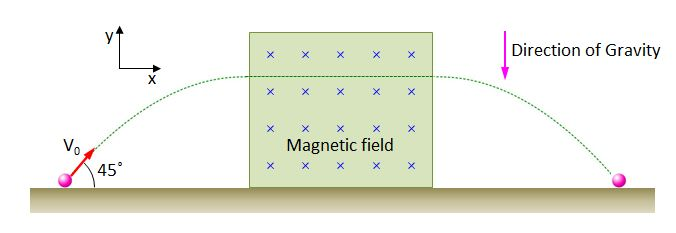# Projectile Motion Of Charged Particle 2The above shows the path of a charged particle with quantity of electricity $+q$ and a mass of $m$ in an $xy$-plane. When the particle is thrown at a $45°$ angle to the $+x$ direction at a speed of $v_0$, its path is a projectile motion initially, and then shows a uniform motion after entering into the uniform magnetic field. Finally, the path becomes a projectile motion again after coming out of the the magnetic field.

What is the intensity of the magnetic field?

Gravitational acceleration is $g$, and the amount of air resistance is negligible.

×

Problem Loading...

Note Loading...

Set Loading...